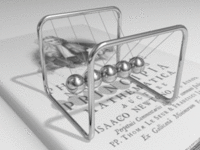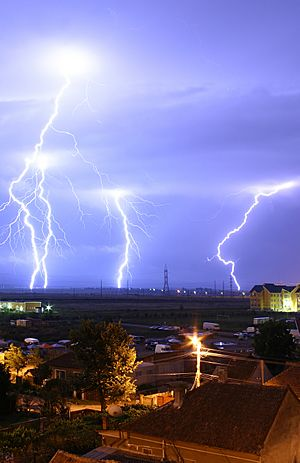# Electrical conductivity facts for kids

Kids Encyclopedia Facts

Electrical conductivity is the measure of a material's ability to allow the transport of an electric charge. Its SI is the siemens per metre, (A2s3m−3kg−1) (named after Werner von Siemens) or, more simply, Sm−1.

It is the ratio of the current density to the electric field strength. It is equivalent to the electrical conductance measured between opposite faces of a 1-metre cube of the material under test.

• The symbol for electrical conductivity is κ (kappa), and also ς (sigma) or γ (gamma).

Electrical conductance is an electrical phenomenon where a material contains movable particles with electric charge (such as electrons), which can carry electricity. When a difference of electrical potential is placed across a conductor, its electrons flow, and an electric current appears.

A conductor such as a metal has high conductivity, and an insulator like glass or a vacuum has low conductivity. A semiconductor has a conductivity that varies widely under different conditions.

Electrical conductivity is the reciprocal (or inverse) of electrical resistivity.

## Causes of conductivity

### Band theory simplified

According to elementary quantum mechanics, electrons in an atom do not take on arbitrary energy values. Rather, electrons only occupy certain discrete energy levels in an atom or crystal; energies between these levels are impossible. When a large number of such allowed energy levels are spaced close together (in energy-space)—i.e. have similar (minutely differing) energies— we can talk about these energy levels together as an "energy band". There can be many such energy bands in a material, depending on the atomic number {number of electrons (if the atom is neutral)} and their distribution (besides external factors like environmental modification of the energy bands).

The material's electrons seek to minimize the total energy in the material by going to low energy states; however, the Pauli exclusion principle means that they cannot all go to the lowest state. The electrons instead "fill up" the band structure starting from the bottom. The characteristic energy level up to which the electrons have filled is called the Fermi level. The position of the Fermi level with respect to the band structure is very important for electrical conduction: only electrons in energy levels near the Fermi level are free to move around, since the electrons can easily jump among the partially occupied states in that region. In contrast, the low energy states are rigidly filled with a fixed number of electrons at all times, and the high energy states are empty of electrons at all times.

### In metalsLike balls in a Newton's cradle, electrons in a metal quickly transfer energy from one terminal to another, despite their own negligible movement.

A metal consists of a lattice of atoms, each with an outer shell of electrons that freely dissociate from their parent atoms and travel through the lattice. This is also known as a positive ionic lattice. This 'sea' of dissociable electrons allows the metal to conduct electric current. When an electrical potential difference (a voltage) is applied across the metal, the resulting electric field causes electrons to drift towards the positive terminal. The actual drift velocity of electrons is very small, in the order of magnitude of a meter per hour. However, as the electrons are densely packed in the material, the electromagnetic field is propagated through the metal at nearly the speed of light. The mechanism is similar to transfer of momentum of balls in a Newton's cradle.

Most metals have resistance. In simpler models (non quantum mechanical models) this can be explained by replacing electrons and the crystal lattice by a wave-like structure each. When the electron wave travels through the lattice the waves interfere, which causes resistance. The more regular the lattice is the less disturbance happens and thus resistance lowers. The amount of resistance is thus caused by mainly two factors. Firstly it is caused by the temperature and thus speed of vibration of the crystal lattice. The temperature causes irregularities in the lattice. Secondly the impurity of the metal is relevant as different ions cause irregularities too.

The larger the cross-sectional area of the conductor, the more electrons per unit length are available to carry the current. As a result, the resistance is lower in larger cross-section conductors. The number of scattering events encountered by an electron passing through a material is proportional to the length of the conductor. The longer the conductor, therefore, the higher the resistance. Different materials also affect the resistance.

### In semiconductors and insulators

In metals, the Fermi level lies in the conduction band (see Band Theory, above) giving rise to free conduction electrons. However, in semiconductors the position of the Fermi level is within the band gap, approximately halfway between the conduction band minimum and valence band maximum for intrinsic (undoped) semiconductors. This means that at 0 kelvin, there are no free conduction electrons, and the resistance is infinite. However, the resistance continues to decrease as the charge carrier density in the conduction band increases. In extrinsic (doped) semiconductors, dopant atoms increase the majority charge carrier concentration by donating electrons to the conduction band or producing holes in the valence band. For both types of donor or acceptor atoms, increasing dopant density reduces resistance. Hence, highly doped semiconductors behave metallically. At very high temperatures, the contribution of thermally generated carriers dominate over the contribution from dopant atoms, and the resistance decreases exponentially with temperature.

### In ionic liquids/electrolytes

In electrolytes, electrical conduction happens not by band electrons or holes, but by full atomic species (ions) traveling, each carrying an electrical charge. The resistivity of ionic solutions (electrolytes) varies tremendously with concentration – while distilled water is almost an insulator, salt water is a reasonable electrical conductor. Conduction in ionic liquids is also controlled by the movement of ions, but here we are talking about molten salts rather than solvated ions. In biological membranes, currents are carried by ionic salts. Small holes in cell membranes, called ion channels, are selective to specific ions and determine the membrane resistance.

### Superconductivity

The electrical resistivity of a metallic conductor decreases gradually as temperature is lowered. In ordinary conductors, such as copper or silver, this decrease is limited by impurities and other defects. Even near absolute zero, a real sample of a normal conductor shows some resistance. In a superconductor, the resistance drops abruptly to zero when the material is cooled below its critical temperature. An electric current flowing in a loop of superconducting wire can persist indefinitely with no power source.

In 1986, researchers discovered that some cuprate-perovskite ceramic materials have much higher critical temperatures, and in 1987 one was produced with a critical temperature above 90 K (−183 °C). Such a high transition temperature is theoretically impossible for a conventional superconductor, so the researchers named these conductors high-temperature superconductors. Liquid nitrogen boils at 77 K, facilitating many experiments and applications that are less practical at lower temperatures. In conventional superconductors, electrons are held together in pairs by an attraction mediated by lattice phonons. The best available model of high-temperature superconductivity is still somewhat crude. There is a hypothesis that electron pairing in high-temperature superconductors is mediated by short-range spin waves known as paramagnons.

### PlasmaLightning is an example of plasma present at Earth's surface. Typically, lightning discharges 30,000 amperes at up to 100 million volts, and emits light, radio waves, and X-rays. Plasma temperatures in lightning can approach 28,000 Kelvin (28,000 °C) (50,000 °F) and electron densities may exceed 1024 m−3.

Plasmas are very good electrical conductors and electric potentials play an important role. The potential as it exists on average in the space between charged particles, independent of the question of how it can be measured, is called the plasma potential, or space potential. If an electrode is inserted into a plasma, its potential generally lies considerably below the plasma potential, due to what is termed a Debye sheath. The good electrical conductivity of plasmas makes their electric fields very small. This results in the important concept of quasineutrality, which says the density of negative charges is approximately equal to the density of positive charges over large volumes of the plasma (ne = <Z>ni), but on the scale of the Debye length there can be charge imbalance. In the special case that double layers are formed, the charge separation can extend some tens of Debye lengths.

In astrophysical plasmas, Debye screening prevents electric fields from directly affecting the plasma over large distances, i.e., greater than the Debye length. However, the existence of charged particles causes the plasma to generate, and be affected by, magnetic fields. This can and does cause extremely complex behavior, such as the generation of plasma double layers, an object that separates charge over a few tens of Debye lengths. The dynamics of plasmas interacting with external and self-generated magnetic fields are studied in the academic discipline of magnetohydrodynamics.Electrical conductivity Facts for Kids. Kiddle Encyclopedia.